Here we will learn about adding and subtracting decimals, including calculations with two or more decimals, or with a mixture of decimals and whole numbers.

Students will first learn about adding and subtracting decimals as part of number and operations in base ten in 5th grade.

## What is adding and subtracting decimals?

Adding and subtracting decimals involves the addition and subtraction of decimal numbers by understanding place value.

When adding or subtracting with decimals special care must be taken to ensure that the decimal points line up with each other. This means that each place value should also line up.

For example, let’s look at 12.5 + 6.23.

Decimal numbers are used in real life particularly when using measurements such as money, length, mass, and capacity. Therefore you may find the skill of adding and subtracting decimals useful when you are problem solving or answering word problems in a real-world context.

On this page, we will be focusing on using the standard algorithm to add or subtract decimals to the thousandths place. No calculations will involve negative numbers or recurring decimals. For information on calculating with negative numbers and different types of decimal numbers, you can follow these links.

Step-by-step guide: Adding and subtracting negative numbers

Step-by-step guide: Recurring decimals

### What is adding and subtracting decimals?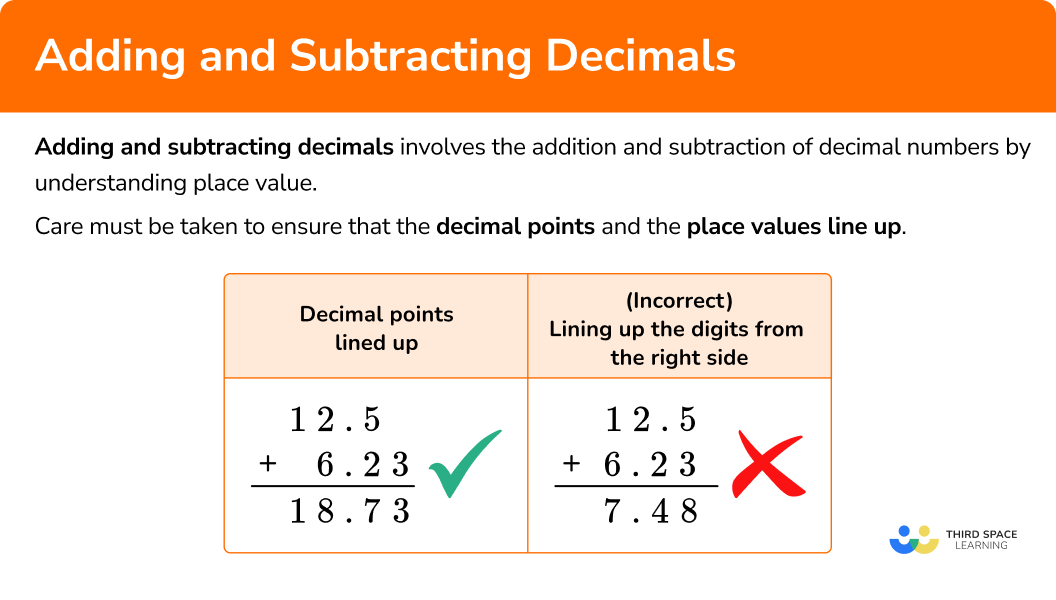## Common Core State Standards

How does this relate to 5th grade math and 6th grade math?

• 5th grade – Numbers and Operations in Base Ten (5.NBT.7)
Add, subtract, multiply, and divide decimals to hundredths, using concrete models or drawings and strategies based on place value, properties of operations, and/or the relationship between addition and subtraction; relate the strategy to a written method and explain the reasoning used

• 6th grade – The Number System (6.NS.3)
Fluently add, subtract, multiply, and divide multi-digit decimals using the standard algorithm for each operation.

## How to add and subtract decimals

In order to add or subtract decimals:

1. Make sure each number has a decimal point and write any \bf{0} placeholders that are required.
2. Stack the numbers, ensuring that the decimal points line up.
3. Use the standard algorithm for addition/subtraction, ensuring the decimal point is also written in the answer.

## Adding and subtracting decimals examples

### Example 1: adding two decimals using the standard algorithm with no regrouping

Calculate 12.3 + 4.5.

1. Make sure each number has a decimal point and write any 0 placeholders that are required.

Each number has a decimal point and one decimal place, so no zero placeholders are required.

2Stack the numbers, ensuring that the decimal points line up.

3Use the standard algorithm for addition/subtraction, ensuring the decimal point is also written in the answer.

Adding the digits in each place value from right to left, we have

Note that the decimal point is placed in the same column in the solution.

So 12.3 + 4.5 = 16.8.

### Example 2: adding a whole number and a decimal using the standard algorithm with no regrouping

Calculate 52 + 31.07.

Make sure each number has a decimal point and write any 0 placeholders that are required.

Stack the numbers, ensuring that the decimal points line up.

Use the standard algorithm for addition/subtraction, ensuring the decimal point is also written in the answer.

### Example 3: adding two decimals using the standard algorithm with regrouping

Calculate 6.7 + 9.31.

Make sure each number has a decimal point and write any 0 placeholders that are required.

Stack the numbers, ensuring that the decimal points line up.

Use the standard algorithm for addition/subtraction, ensuring the decimal point is also written in the answer.

### Example 4: subtracting two decimals using the standard algorithm with no regrouping

Calculate 26.87-14.2.

Make sure each number has a decimal point and write any 0 placeholders that are required.

Stack the numbers, ensuring that the decimal points line up.

Use the standard algorithm for addition/subtraction, ensuring the decimal point is also written in the answer.

### Example 5: subtracting a decimal from a whole number using the standard algorithm with regrouping

Calculate 16-9.4.

Make sure each number has a decimal point and write any 0 placeholders that are required.

Stack the numbers, ensuring that the decimal points line up.

Use the standard algorithm for addition/subtraction, ensuring the decimal point is also written in the answer.

### Example 6: subtracting two decimals using the standard algorithm with regrouping

Calculate 2.04-0.952.

Make sure each number has a decimal point and write any 0 placeholders that are required.

Stack the numbers, ensuring that the decimal points line up.

Use the standard algorithm for addition/subtraction, ensuring the decimal point is also written in the answer.

### Teaching tips for adding and subtracting decimals

• Review place values before beginning to add or subtract decimals, especially decimal place values. Not only will students need to line up the decimal point, but they also need to ensure that each place value is lined up, so their understanding of place values is vital.

• Students may struggle to line up the digits when stacking the numbers, so it may help to provide them with graph paper so they may write the numbers into boxes and keep them aligned. This will also help students see what place values are “missing” a number, and they can add in a zero placeholder into that box.

• Provide students with opportunities to solve real-world word problems involving adding or subtracting decimals, such as money or measurement. This will help them better understand the problems and what the numbers represent in a real-life context.

### Easy mistakes to make

• Lining up decimal numbers in each place value incorrectly
When using the standard algorithm for addition of decimals or subtraction of decimals, students can sometimes line up the numbers incorrectly. This is because younger students are sometimes told to line up the numbers from the right side, but this method only works for whole numbers.

When stacking the decimal numbers, you must line up the decimal points. This will ensure that the digits are in the correct column according to their place value. Using zero placeholders can also help you to avoid making this mistake.

• Subtracting the smaller digit from the larger digit during standard algorithm subtraction even though the smaller digit is above the larger digit
When using the standard algorithm for decimal subtraction, students can find themselves automatically subtracting the smaller digit from the larger digit, rather than taking time to note which digit is supposed to be subtracted from the other. The digit below should always be subtracted from the digit above.

If the digit above is smaller than the digit below then a method often referred to as ‘borrowing’ must take place. For instance, here is the incorrect method for example 5 where the smaller digit which is above has been incorrectly subtracted from the larger digit. Also see the correct method where ‘borrowing’ has taken place.

Incorrect solution:

Correct solution:

• Forgetting to write the decimal point in the solution to a standard algorithm addition/subtraction problem involving decimals
When using the standard algorithm for addition or subtraction of decimals, students can sometimes forget to write the decimal point in their solution. It is important to remember to write this, particularly if the answer is not a whole number. It is good practice to write the decimal point in the solution area before you start to solve. The decimal point should line up with the points above.

Note how the decimal point has been written in the solution area before the calculation has been started.

### Practice adding and subtracting decimals questions

1. Solve 58.1 + 0.46.

62.758.566.275.856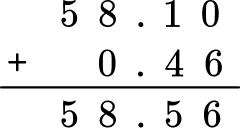2. Solve 41.3 + 38.

79.341.6841.4145.1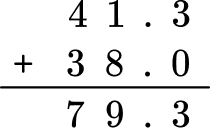3. Solve 10.62 + 7.73.

17.2317.13518.3587.92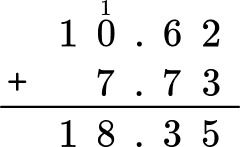4. Solve 16.9-3.3.

13.616.5713.871.36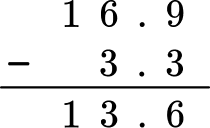5. Solve 27-1.24.

26.2425.7614.61.46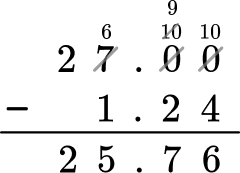6. Solve 7.11-6.84.

64.261.731.950.27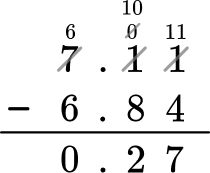## Adding and subtracting decimals word problems

1. This table shows the 4 most recent world records for the men’s 100 meter race.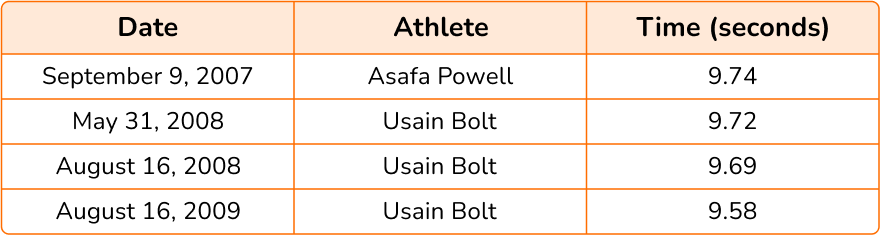Usain Bolt holds the current world record for the men’s 100 meter race at 9.58 seconds.

How many seconds did he shave off the previous world record holder’s time?

9.74-9.58 = 0.16 seconds

2. Abi, Bobby and Cyrus each have some money.

They want to buy a ball from a local shop costing \$3.60 to play catch with. They decide to put their money together in order to buy the ball. Abi has \$2.30.

Bobby has \$1.25. Cyrus has 9 cents. If they buy the ball, how much change will they get? Show answer 2.30 + 1.25 + 0.09 = 3.64 3.64-3.60 = 0.04 Change is \$0.04 or 4 cents.

3. Ali is harvesting potatoes. He weighs and measures the length of a sample of 10 potatoes. Below is a table showing his results.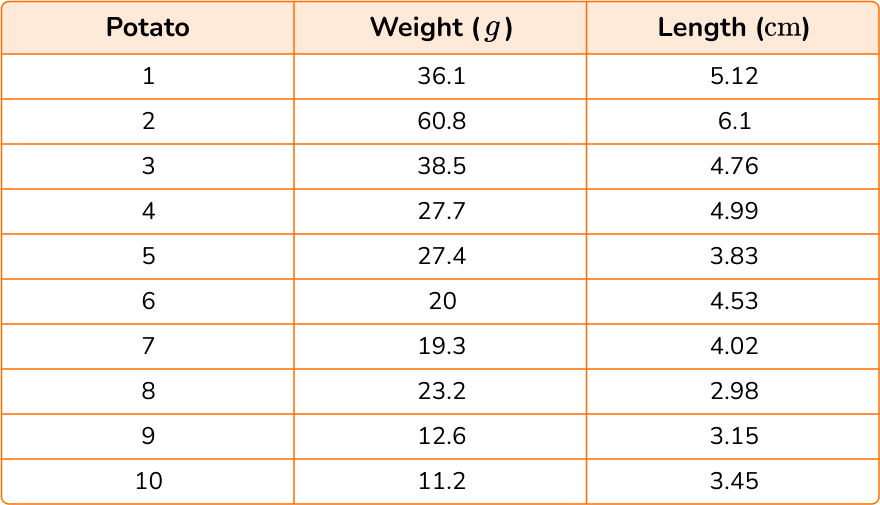(a) Find the difference between the longest potato and the shortest potato in the sample.

(b) What is the total weight of the 3 longest potatoes?

(a) Longest shortest = 6.1-2.98 = 3.12 \, cm

(b) Potatoes 1, 2, and 4\text{: } 36.1 + 60.8 + 27.7 = 124.6 \, g

## Adding and subtracting decimals FAQs

What is the first step in adding and subtracting decimals?

The first step is to stack the numbers, lining up the numbers according to place value and lining up the decimal points.

How can you add or subtract decimals if the numbers do not have the same number of digits in the decimal places?

To add or subtract decimals that do not have the same number of digits in the decimal places, you can use zeros as placeholders and then begin to solve.

Where do you place the decimal point in the answer when you add or subtract decimals?

In the answer, the decimal point should line up with the decimal points in the numbers you are adding or subtracting. It may be helpful to place the decimal point in the answer space first before beginning to solve.

## Still stuck?

At Third Space Learning, we specialize in helping teachers and school leaders to provide personalized math support for more of their students through high-quality, online one-on-one math tutoring delivered by subject experts.

Each week, our tutors support thousands of students who are at risk of not meeting their grade-level expectations, and help accelerate their progress and boost their confidence.